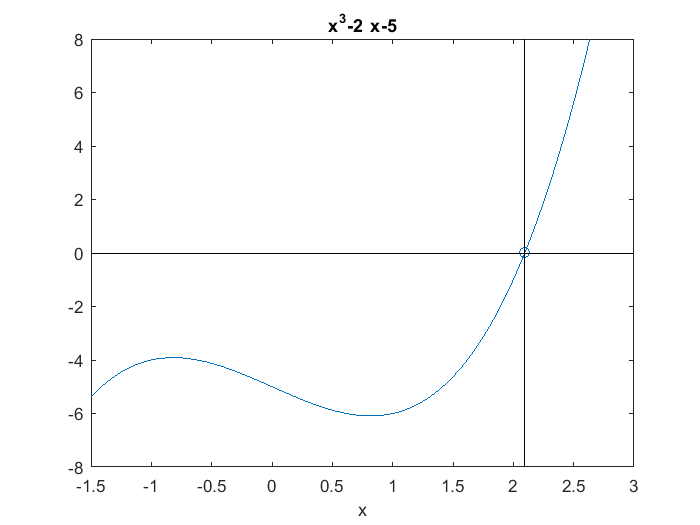# A Historic Cubic

The cubic polynomial $x^3 - 2x - 5$ has a unique place in the history of numerical methods.

### Contents

#### Whittaker and Robinson

The Calculus of Observations, A Treatise on Numerical Mathematics, by E. T. Whittaker and G. Robinson, is a classic text on numerical analysis that was first published in London in 1924. A scan of the complete book, made by the Million Books Project, is available online at the link referenced below. (The copy of the book that was scanned is actually from India, the library of Osmania University in Hyderabad.)

The section on the Newton-Raphson method uses

$$x^3-2x-5$$

as the first example. A footnote on the example caught my eye years ago and I've always remembered it.

#### The footnote

"The reason I call $x^3-2x-5=0$ a celebrated equation is because it was the one on which Wallis chanced to exhibit Newton's method when he first published it, in consequence of which every numerical solver has felt bound in duty to make it one of his examples. Invent a numerical method, neglect to show how it works on this equation, and you are a pilgrim who does not come in at the little wicket (vide J. Bunyan)."

#### De Morgan

Augustus de Morgan was an eminent 19-th century British mathematician. He formulated De Morgan's laws of boolean algebra and propositionai logic, and was known as a witty correspondent. The footnote in Whittaker and Robinson is a quotation from one of many letters that de Morgan wrote to his friend and former teacher at Trinity College, Cambridge, William Whewell. The letter was written January 20th, 1861, and is included in a memoir of de Morgan published by his wife, Sophia Elizabeth, in 1882. A scan of the memoir, made by the Internet Archives project, is also available online. (The copy of the book came from the library at the University of California, Santa Cruz.)

#### Wicket Gate

I've never seen or heard the phrase "a pilgrim who does not come in at the little wicket" anyplace else. If any reader of this blog can tell us more about it, please contribute a comment.

A wicket gate is a small gate, often part of a larger one. The Pilgrim referred to is the protagonist Christian in Pilgrim's Progress, a 17-th century allegory by John Bunyan. Many of us remember Pilgrim's Progress from our studies of English literature. Christian entering the Wicket Gate is analogous to Everyman obtaining Salvation.Christian enters the Wicket Gate in Pilgrim's Progress

http://en.wikipedia.org/wiki/The_Pilgrim's_Progress

#### Cardano's formula

Most of us also remember the formula for solving a quadratic equation. There is a corresponding formula for solving cubics, but I bet that few of my readers can spontaneously recite it.

Gerolamo Cardano and Niccolo Fontana Tartaglia were 16-th century Italian polymaths who had what was at first a friendly competition and later a famous feud about how to solve cubic equations. They probably both learned how to solve them from the work of another Italian, Scipione del Ferro.

What we now know as Cardano's formula applies to a depressed cubic where the quadratic term has been removed by a simple change of variable. A depressed cubic equation is

$$x^3 = px + q$$

Cardano's formula for the solution is

$$x = \sqrt{\frac{q}{2}+\sqrt{\frac{q^2}{4}-\frac{p^3}{27}}} + \sqrt{\frac{q}{2}-\sqrt{\frac{q^2}{4}-\frac{p^3}{27}}}$$

My favorite cubic is already depressed, so

   p = 2;
q = 5;
s = sqrt(q^2/4 - p^3/27);
x0 = (q/2 + s)^(1/3) + (q/2 - s)^(1/3)

x0 =
2.094551481542327


#### ezplot

Here is the interesting portion of the graph of our cubic with the axes through the solution obtained from Cardano's formula.

   f = @(x) x.^3-2*x-5
ezplot(f,[-1.5 3])
axis([-1.5 3 -8 8])
line([x0 x0],[0 0],'marker','o')
line([x0 x0],[-8 8],'color','k')
line([-1.5 3],[0 0],'color','k')

f =
@(x)x.^3-2*x-5#### Seeking Salvation

Cardano's formula applies only to cubics. So in my next post I plan to try out several different algorithms that apply to more general equations and confirm that their MATLAB implementations come in at de Morgan's little wicket.

#### References

E. T. Whittaker and G. Robinson, The Calculus of Observations, A Treatise on Numerical Mathematics. Blackie and Son Limited, London, 1924, 394 pp. <http://archive.org/details/calculusofobserv031400mbp>

Sophia Elizabeth De Morgan, Memoir of August De Morgan. Longmans, Green, and Co., London, 1882, 468 pp. <http://archive.org/details/memoirofaugustus00demorich>

Published with MATLAB® R2015a

|